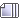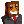Author Topic:  Bump..  (Read 35314 times)

0 Members and 1 Guest are viewing this topic.

RockRe: Bump.
« Reply #10 on: September 14, 2015, 05:20:50 PM »
Butt = Bump up this topic, but if the topic's yours...
Bumt = Bump up my topic.
hi

XdeathXreaperX1

• GuestRe: Bump.
« Reply #11 on: September 14, 2015, 05:27:08 PM »
OP updated

HoundeyeRe: Bump.
« Reply #12 on: September 14, 2015, 05:36:05 PM »
Smack.

II FerGie x 🍀Re: Bump.
« Reply #13 on: September 14, 2015, 05:47:26 PM »
You guys aren't spamming this topic enough, I'm seriously disappointanted in you all. :-/JesseRe: Bump.
« Reply #14 on: September 14, 2015, 05:55:38 PM »
Bump

You guys aren't spamming this topic enough, I'm seriously disappointanted in you all. :-/
Would like me to?Thanks GK for SigMotM September '14Robo February '154th Demi Gods December '16YouTube: Mythical MonkeyNeftyRe: Bump.
« Reply #15 on: September 14, 2015, 06:03:42 PM »
Bump

An illustration of a bump function in two variables.
In mathematics, a bump function is a function f : Rn → R on a Euclidean space Rn which is both smooth (in the sense of having continuous derivatives of all orders) and compactly supported. The space of all bump functions on Rn is denoted C^\infty_0(\mathbf{R}^n) or C^\infty_c(\mathbf{R}^n). The dual space of this space endowed with a suitable topology is the space of distributions.

Contents  [hide]
1 Examples
2 Existence of bump functions
3 Properties and uses
5 References

Examples

The function Ψ(x).
The function Ψ : R → R given by
\Psi(x) = \begin{cases} e^{ -\frac{1}{1 - x^2}} & \mbox{ for } |x| < 1\\ 0 & \mbox{ otherwise} \end{cases}
is an example of a bump function in one dimension. It is clear from the construction that this function has compact support, since a function of the real line has compact support if and only if it has bounded support. The proof of smoothness follows along the same lines as for the related function discussed in the Non-analytic smooth function article. This function can be interpreted as the Gaussian function e^{-y^2} scaled to fit into the unit disc: the substitution y^2=1/(1-x^2) corresponds to sending x = ±1 to y = ∞.

A simple example of a bump function in n variables is obtained by taking the product of n copies of the above bump function in one variable, so
\Phi(x_1, x_2, \dots, x_n) = \Psi(x_1)\Psi(x_2)\cdots\Psi(x_n).
Existence of bump functions

An illustration of the sets in the construction.
It is possible to construct bump functions "to specifications". Stated formally, if K is an arbitrary compact set in n dimensions and U is an open set containing K, there exists a bump function φ which is 1 on K and 0 outside of U. Since U can be taken to be a very small neighborhood of K, this amounts to being able to construct a function that is 1 on K and falls off rapidly to 0 outside of K, while still being smooth.

The construction proceeds as follows. One considers a compact neighborhood V of K contained in U, so K ⊂ Vo ⊂ V ⊂ U. The characteristic function \chi_V of V will be equal to 1 on V and 0 outside of V, so in particular, it will be 1 on K and 0 outside of U. This function is not smooth however. The key idea is to smooth \chi_V a bit, by taking the convolution of \chi_V with a mollifier. The latter is just a bump function with a very small support and whose integral is 1. Such a mollifier can be obtained, for example, by taking the bump function \Phi from the previous section and performing appropriate scalings.

Properties and uses

While bump functions are smooth, they cannot be analytic unless they vanish identically. This is a simple consequence of the identity theorem.

Bump functions are often used as mollifiers, as smooth cutoff functions, and to form smooth partitions of unity. They are the most common class of test functions used in analysis.

The space of bump functions is closed under many operations. For instance, the sum, product, or convolution of two bump functions is again a bump function, and any differential operator with smooth coefficients, when applied to a bump function, will produce another bump function.

The Fourier transform of a bump function is a (real) analytic function, and it can be extended to the whole complex plane: hence it cannot be compactly supported unless it is zero, since the only entire analytic bump function is the zero function (see PaleyWiener theorem). Because the bump function is infinitely differentiable, its Fourier transform F(k) must decay faster than any finite power of 1/k for a large angular frequency |k|. The Fourier transform of the particular bump function
\Psi(x) = e^{-\frac{1}{1-x^2}} \mathbf{1}_{\{|x|<1\}}
from above can be analyzed by a saddle-point method, and decays asymptotically as
|k|^{-\frac{3}{4}} e^{-\sqrt{|k|}}
for large |k|.

As many W's as possibleRe: Bump.
« Reply #16 on: September 14, 2015, 06:06:11 PM »
+1 Nefty. Bump.

NeftyRe: Bump.
« Reply #17 on: September 14, 2015, 06:11:45 PM »
+1 Nefty. Bump.

But what does it truly mean to be bumped? Aristotle belied in an objective form of ethics, but Kant disagreed because it bumped into his idea of self morality. But is that truly what it means Bump. It will be glorious day when we no longer have to live in the shadows wondering what it truly means, to bump.

As many W's as possibleRe: Bump.
« Reply #18 on: September 14, 2015, 06:13:30 PM »
+1 Nice Post. Bump.

Edit: OP Updated.
« Last Edit: September 14, 2015, 06:15:22 PM by Tsar Trubtavium VI ☭ »

RockRe: Bump.
« Reply #19 on: September 14, 2015, 06:24:38 PM »
Bump
hi